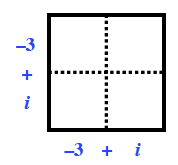Home > CCA2 > Chapter 8 > Lesson 8.2.1 > Problem8-72

8-72.
1. If f(x) = x2 + 7x − 9, calculate the values in parts (a) through (c) below. Homework Help ✎

1. f(−3)

2. f(i)

3. f(−3 + i)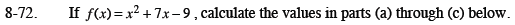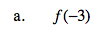f(−3) = −21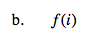f(i) = i2 + 7i − 9

f(i) = −10 + 7i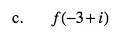$f(-3+ i)= (-3+i)^2+7(-3 + i) - 9$

Use a generic rectangle to expand (−3 + i)2.